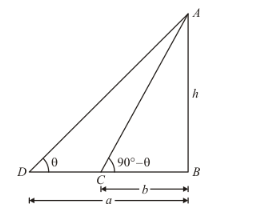# If the angles of elevation of the top of a tower from two points distant a and b from

Question:

If the angles of elevation of the top of a tower from two points distant a and b from the  base and in the same straight line with it are complementary, then the height of the tower is

(a) $a b$

(b) $\sqrt{a b}$

(c) $\frac{a}{b}$

(d) $\sqrt{\frac{a}{b}}$

Solution:

Letbe the height of tower.Given that: angle of elevation of top of the tower areand.

DistanceandHere, we have to find the height of tower.

So we use trigonometric ratios.

In a triangle,

$\Rightarrow \tan C=\frac{A B}{B C}$

$\Rightarrow \tan \left(90^{\circ}-\theta\right)=\frac{h}{h}$

$\Rightarrow \cot \theta=\frac{h}{b}$

Again in a triangle ABD,

$\tan D=\frac{A B}{B D}$

$\Rightarrow \tan \theta=\frac{h}{a}$

$\Rightarrow \frac{1}{\cot \theta}=\frac{h}{a}$

$\Rightarrow \frac{b}{h}=\frac{h}{a}$

$\Rightarrow h^{2}=a b$

$\Rightarrow h=\sqrt{a b}$

Put $\cot \theta=\frac{h}{b}$

Hence the correct option is $b$.